# An unequal pyramid

Last post, I worked on the vertex edge angles of a triangular pyramid that had all equal angles originating from the apex.

Using the same camera tripod analogy, take one of the legs (line DA) of the tripod and slide it out further from the other two, but keep it equally distant from each. You now have a camera that’s about to crash to the floor, but you also have a pyramid that has two equal apex angles and that leans.

A right pyramid is a pyramid for which the apex lies directly above the centroid of the base. I don’t know if there is an actual name for a leaning pyramid, but I will just call it a leaning pyramid.

Again, we will work with a pyramid with vertexes A, B, C, & D, with D as the apex (Fig. 1). Again, the legs DA, DB, & DC are all equal length of 1 unit.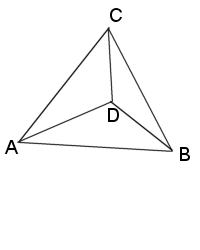Figure 1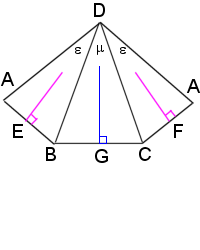Figure 2

We can “unfold” the pyramid (Fig. 2) to show the bisection of the apex angles where they join the base vertexes, the midpoints E, F, & G.

We can see BG=CG, and AE=BE=CF=AF, and DE=DF.

Since ΔDAB = ΔDAC, we will ignore showing the the latter in the formulas. Both are isosceles, as well as ΔDBC.

$$\large{ BG=CG= sin(\frac{\mu}{2})\\ BC=2\cdot sin(\frac{\mu}{2})\\ DG=cos(\frac{\mu}{2})\\ AE=BE= sin(\frac{\epsilon}{2})\\ AB=2\cdot sin(\frac{\epsilon}{2})\\ DE=cos(\frac{\epsilon}{2})}$$

Items that must be true for this to be a real 3D object: $$2\cdot\epsilon>\mu, 2\cdot\epsilon+\mu<360^\circ$$.

Figure 3 shows how to find the line AG on the base triangle. Using the Pythagorean theorem, $$AG=\sqrt{4\cdot sin^{2}(\epsilon/2)-sin^{2}(\mu/2)}$$.

We split the pyramid in half, giving us cross section ΔADG (Fig. 4).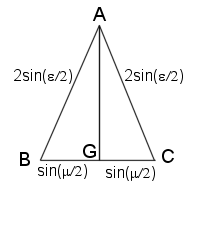Figure 3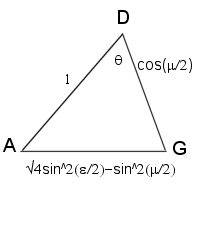Figure 4

Using law of cosines, we get:

\large{\begin{align}\cos\theta &=\frac{1^2+\cos^{2}(\frac{\mu}{2})-4\cdot \sin^{2}(\frac{\epsilon}{2})+\sin^{2}(\frac{\mu}{2})}{2\cdot 1\cdot \cos(\frac{\mu}{2})}\\ &=\frac{1 +[\cos^{2}(\frac{\mu}{2})+\sin^{2}(\frac{\mu}{2})]-4\cdot \sin^{2}(\frac{\epsilon}{2})}{2\cdot \cos(\frac{\mu}{2})} &\text{Rearrange figures}\\ &=\frac{1+-4\cdot \sin^{2}(\frac{\epsilon}{2})}{2\cdot \cos(\frac{\mu}{2})} &\text{cos²α + sin²α = 1}\\ &=\frac{2-4\cdot \sin^{2}(\frac{\epsilon}{2})}{2\cdot \cos(\frac{\mu}{2})}\\ &=\frac{1-2\cdot \sin^{2}(\frac{\epsilon}{2})}{\cos(\frac{\mu}{2})}\\ &=\frac{\cos(\epsilon)}{\cos(\frac{\mu}{2})} & \text{1-2sin²α = cos 2α} \end{align}}

We can now use this new formula to double check our work on the truncated icosahedron. Hexagons have exterior angles of 120° (ε) and pentagons are 108° (μ). So we get:

$$cos\theta=\frac{\cos(120)}{\cos(\frac{108}{2})}=\frac{cos(120)}{cos(54)}\\ \theta=148.2825256\ldots^\circ$$

The formula can be used to quickly find the vertex edge angle of any of the Platonic or Archimedean solids that have only 3 shapes meeting at each vertex, with 2 (or all 3) of them the same. (Although it isn’t as much fun as actually working it out ourselves.) It can also be used on prisms, but that is trivial, as the edge will always be at 90° to the bases.

We can find the angle made by slicing the pyramid through ΔCDE, but the result really doesn’t mean much as the plane made by ΔCDE is not perpendicular to the plane made by the base. ΔADG is perpendicular to the base plane. There would be 2 angles needed to describe the result. Example, looking at the truncated icosahedron, you can see the edges splaying out from the pentagons even with the center lines, sort of like the spokes of a wheel, but looking at the hexagons, the edges don’t line up with the center lines, they are slightly off.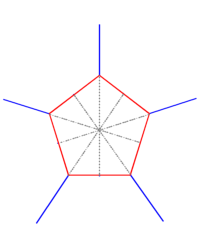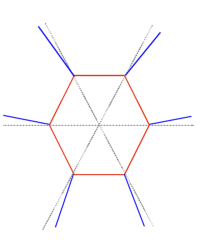Likewise, in a pyramid with all 3 apex angles different, there would be no cross section that was perpendicular to the base plane, so no real usage here.

# Tetrahedron

I started off posting about the truncated icosahedron, despite being fairly complex compared to the platonic solids, like the tetrahedron.

The regular tetrahedron is probably the simplest 3D shape, except for maybe the sphere. It is made of 4 equilateral triangles, forming a triangular pyramid.

Starting with a tetrahedron with vertexes named A, B, C, & D, with D as the apex (fig. 1), we can bisect ΔABC from point A to line BC and call that point G (fig. 2).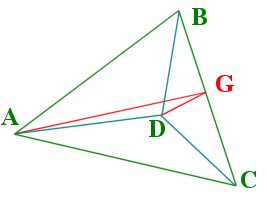Figure 1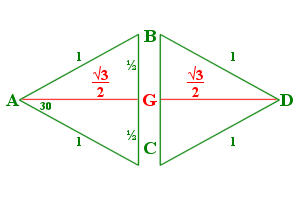Figure 2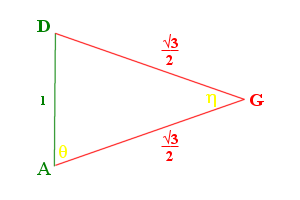Figure 3

The bisection of ΔDBC would follow the same, a line DG would also equal AG. Figure 3 shows the cross section of the tetrahedron, ΔADG. We can then use the law of cosines to find ∠θ and ∠η.

$$\Large\cos \eta = \frac{AG^{2} + DG^{2} – AD^{2}}{2\cdot AG \cdot DG} = \frac{\frac34 + \frac34 – 1}{2\cdot\frac{\sqrt{3}}{2}\cdot\frac{\sqrt{3}}{2}} = \frac{\frac24}{\frac64} = \frac13$$.

The angle (η) between each face, the dihedral angle, is $$cos^{-1} (\frac{1}{3})=70.528779\ldots^\circ$$.

$$\Large\cos \theta = \frac{AG^{2} + AD^{2} – DG^{2}}{2\cdot AG \cdot AD} = \frac{\frac34 + 1 – \frac34}{2\cdot 1\cdot\frac{\sqrt{3}}{2}} = \frac{1}{\sqrt3}$$.

The angle that the edge to face (θ) makes would be $$cos^{-1}\frac{1}{\sqrt{3}}= 54.73561\ldots^\circ$$. Also since ΔADG is isosceles, ∠ADG = ∠DAG = θ.

$$2\cdot\theta+\eta=180^\circ$$

From the above equations and figures, you can then calculate other aspects, such as the height.

But what about non-regular tetrahedra? Let’s think about other pyramids, both fat and short and tall and thin. All the triangles in the regular tetrahedron have three 60° corners.

Let’s suppose that the angles originating at point D are all equal (we will call them λ), and the edges are also equal (we will call them length 1). We would have something like that of a camera tripod.

If we spread the legs, the camera will get lower to the ground and if we bring the legs together, the camera will get higher. The same is true of our pyramid.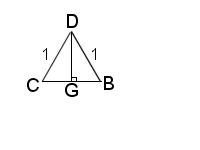Figure 4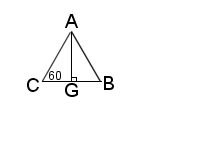Figure 5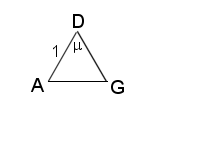Figure 6

Let us again bisect the angles λ, as shown in fig. 4.

$$BG = CG= \sin(\frac{\lambda}{2})$$, $$BC= 2\cdot \sin(\frac{\lambda}{2})$$, $$DG= \cos(\frac{\lambda}{2})$$.

Looking at the pyramid’s base ΔABC (fig. 5), $$BC = AC= AB = 2\cdot \sin(\frac{\lambda}{2})$$, then $$AG = BG\cdot\sqrt{3} = \sqrt{3}\cdot sin(\frac{\lambda}{2})$$.

We again us the law of cosines to find μ (fig. 6).

$$\Large\cos\mu=\frac{1+cos^{2}\frac{\lambda}{2} – 3\cdot sin^{2}\frac{\lambda}{2}}{2\cdot cos\frac{\lambda}{2}}$$

If we add $$(1\cdot sin^{2}\frac{\lambda}{2} -1\cdot sin^{2}\frac{\lambda}{2})$$, which equals zero, to the top of the equation, we get $$\large\cos\mu=\frac{1+(cos^{2}\frac{\lambda}{2}) – 4\cdot sin^{2}\frac{\lambda}{2}+(sin^{2}\frac{\lambda}{2})}{2\cdot cos\frac{\lambda}{2}}$$. Note the change of the three to four.

The items in parentheses can be combined using the identity cos² α + sin² α = 1:

$$\Large\cos\mu=\frac{1+1-4\cdot sin^{2}\frac{\lambda}{2}}{2\cdot cos\frac{\lambda}{2}}=\frac{2-4\cdot sin^{2}\frac{\lambda}{2}}{2\cdot cos\frac{\lambda}{2}}=\frac{1-2\cdot sin^{2}\frac{\lambda}{2}}{cos\frac{\lambda}{2}}$$.

Using the identity cos 2α = 1 – 2·sin² α, gives: $$\large\cos\mu=\frac{cos\lambda}{cos\frac{\lambda}{2}}$$.

We can then use this formula to verify the regular tetrahedron’s vertex edge angle that we found earlier. If $$\lambda=60^\circ$$, $$\large\cos\mu=\frac{cos60}{cos30}=\frac{\frac12}{\frac{\sqrt{3}}{2}}=\frac{1}{\sqrt{3}}$$, which is the same as θ above.

This formula can also be used on other polyhedra where there are three identical angles joining at a vertex, such as the cube,$$\large\cos\mu=\frac{cos90}{cos45}=\frac{0}{\frac{1}{\sqrt2}}=0, \mu=90^\circ$$ or dodecahedron, $$\large\cos\mu=\frac{cos108}{cos54}=\frac{\frac{1-\sqrt5}{4}}{\frac{\sqrt{10-2\sqrt5}}{4}}=\frac{1-\sqrt5}{\sqrt{10-2\sqrt5}}, \mu=121.71747^\circ$$.

I will get to the dodecahedron soon. The cube is so easy to figure out mathematically, rather boring.

Next posting, I will show you what happens if the pyramid leans a bit, that is if one of the apex angles is different that the other two.